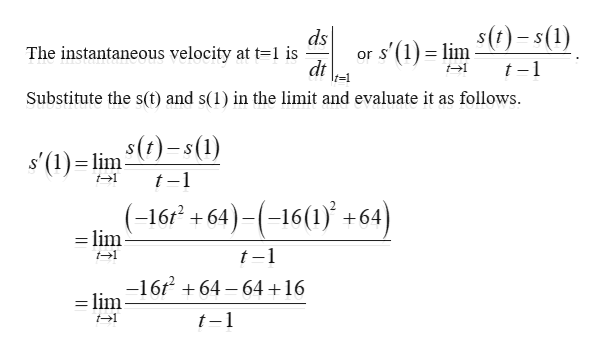# A rock is dropped from a height of 64ft. Its height above ground at time t seconds later is given by s(t)=−16t2+64 for 0≤t≤2. Find its instantaneous velocity 1 second after it is dropped using the limit definition of a derivative. Do not include units in your answer.

Question
8 views

A rock is dropped from a height of 64ft. Its height above ground at time t seconds later is given by s(t)=−16t2+64 for 0≤t≤2. Find its instantaneous velocity 1 second after it is dropped using the limit definition of a derivative. Do not include units in your answer.

check_circle

Step 1

Consider the model function that represents the height above the ground as s(...help_outlineImage Transcriptionclosers'(1) lim )=s(1) dt ds The instantaneous velocity at t=1 is t-1 t=1 Substitute the s(t) and s(1) in the limit and evaluate it as follows \$(1) lim ()-s(1) t1 t-1 .(-16 +64)-(-16(1+64) lim t-1 -16 64 6416 =lim t-1 t1 fullscreen

### Want to see the full answer?

See Solution

#### Want to see this answer and more?

Solutions are written by subject experts who are available 24/7. Questions are typically answered within 1 hour.*

See Solution
*Response times may vary by subject and question.
Tagged in

### Other